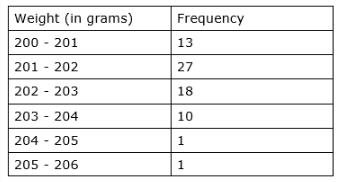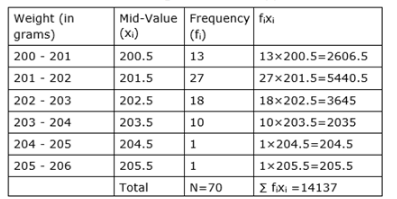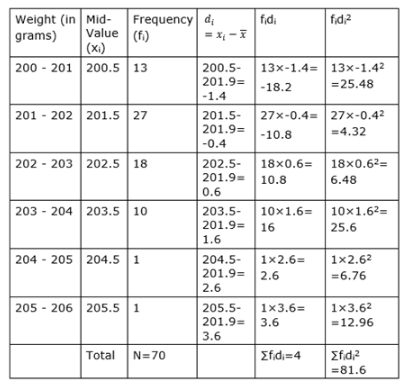# The weights of coffee in 70 jars are shown in the following table:

Question:

The weights of coffee in 70 jars are shown in the following table:Determine variance and standard deviation of the above distribution.

Solution:

Given the weights of coffee in 70 jars

Now we have to find the variance and standard deviation of the distribution

Let us make a table of the given data and append other columns after calculationsHere mean, ${ }^{\bar{x}}=\frac{\sum f_{1} x_{1}}{N}=\frac{14137}{70}=201.9$

So the above table with more columns is as shown below,And we know standard deviation is

$\sigma=\sqrt{\frac{\sum f_{i} d_{i}^{2}}{n}-\left(\frac{\sum f_{i} d_{i}}{n}\right)^{2}}$

Substituting values from above table, we get

$\sigma=\sqrt{\frac{81.6}{70}-\left(\frac{4}{70}\right)^{2}}$

$\sigma=\sqrt{1.17-(0.057)^{2}}$

$\sigma=\sqrt{1.17-0.003249}=\sqrt{1.17}$

$\Rightarrow \sigma=1.08 \mathrm{~g}$

And $\sigma^{2}=1.08^{2}=1.17 \mathrm{~g}$

Hence the variance and standard deviation of the distribution are $1.166 \mathrm{~g}$ and $1.08$ respectively.